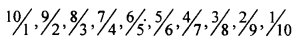# KSEEB Solutions for Class 8 Maths Chapter 7 Rational Numbers Ex 7.2

Students can Download Maths Chapter 7 Rational Numbers Ex 7.2 Questions and Answers, Notes Pdf, KSEEB Solutions for Class 8 Maths helps you to revise the complete Karnataka State Board Syllabus and score more marks in your examinations.

## Karnataka Board Class 8 Maths Chapter 7 Rational Numbers Ex 7.2

Question 1.
Write down ten rational numbers which are equivalent to $$\frac { 5 }{ 7 }$$ and the denominator not exceeding 80.
Multiply both numerator and denominator by 2, 3, 4………
$$\frac{10}{14}, \frac{15}{21}, \frac{20}{28}, \frac{35}{35}, \frac{30}{42}, \frac{35}{49}, \frac{40}{56}, \frac{45}{63}, \frac{50}{70}, \frac{55}{77}$$

Question 2.
Write down 15 rational numbers which are equivalent to $$\frac { 11 }{ 5 }$$ and the numerator not exceeding 180.
$$\begin{array}{l}{\frac{22}{10}, \frac{33}{15}, \frac{44}{20}, \frac{55}{25}, \frac{66}{30}, \frac{77}{35}, \frac{88}{40}, \frac{99}{45}} \\ {\frac{110}{50}, \frac{121}{55}, \frac{132}{60}, \frac{143}{65}, \frac{154}{70}, \frac{165}{75}, \frac{176}{80}}\end{array}$$

Question 3.
Write down 10 positive rational numbers such that the sum of the numerator and the denominator of each is 11. Write them in decreasing order.Question 4.
Write down ten positive rational numbers such that numerator – denominator for each of them is -2. Write to them in increasing order.
Numerator – denominator = – 2
therefore the denominator is greater than the numerator by 2.
$$\frac{1}{3}, \frac{2}{4}, \frac{3}{5}, \frac{4}{6}, \frac{5}{7}, \frac{6}{9}, \frac{7}{9}, \frac{8}{10}, \frac{9}{11}, \frac{10}{12}$$

Question 5.
Is $$\frac { 3 }{ -2 }$$ a rational number? If so, how do you write it in the form conforming to the definition of a rational number (that is, the denominator as positive integer)?
$$\frac{3}{-2}$$ is a rational number because the denominator is negative.
It can be written as $$\frac{3}{-2}$$ since $$\frac{3}{-2}$$ is same as $$\frac{3}{-2}$$
$$0.9=\frac{9}{10} \text { and } 0.8=\frac{8}{10}=\frac{4}{5}$$